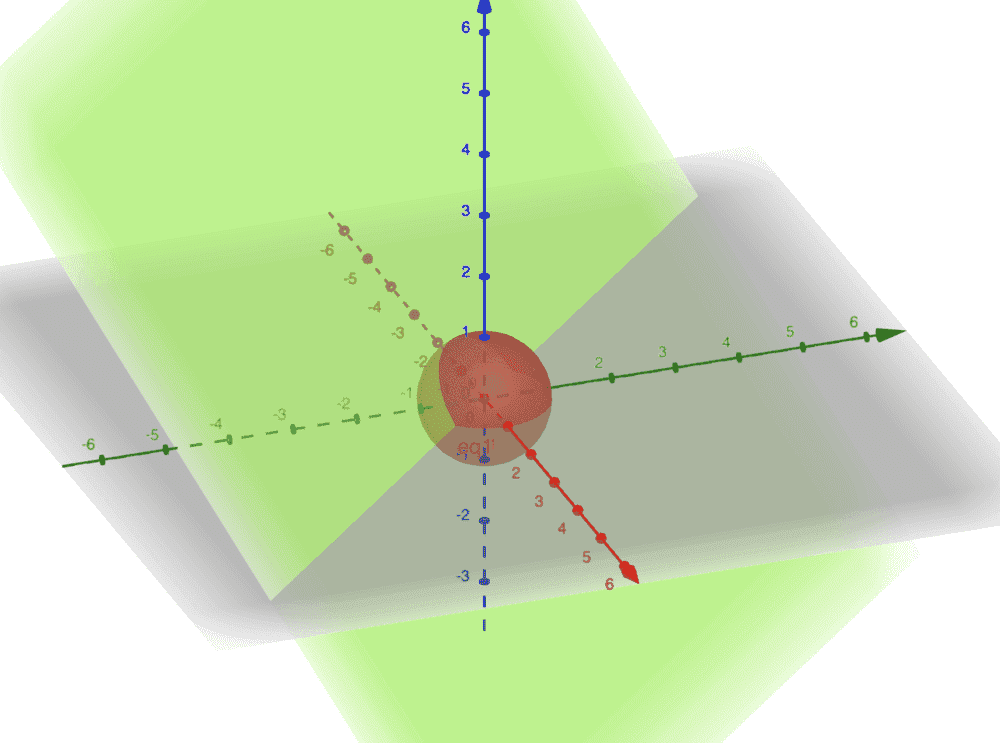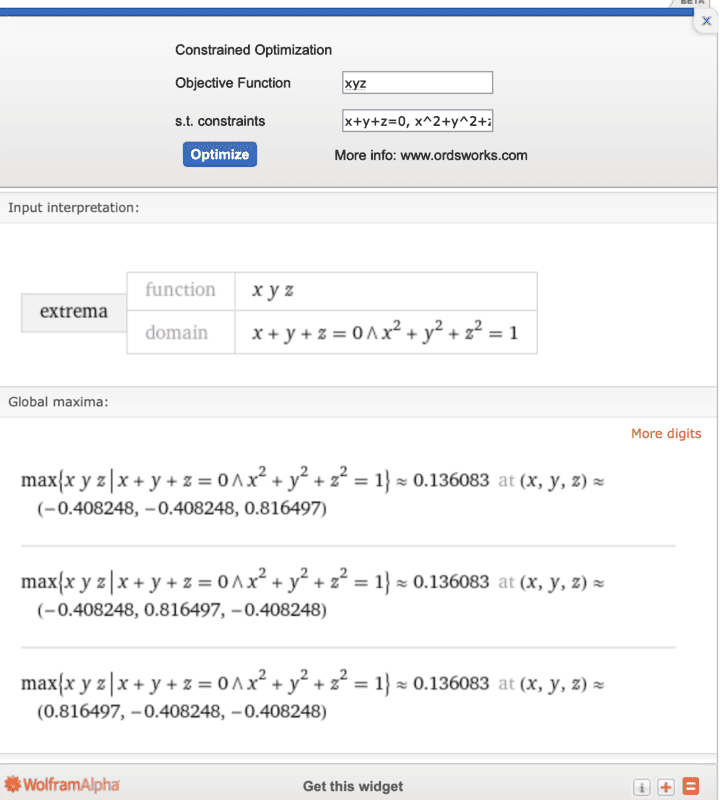# Maximize the function with constraints

• docnet
In summary, the conversation discusses various approaches to solve an optimization problem involving a domain bounded by a circle of radius 1 centered at the origin, and a plane intersecting the sphere. The conversation touches on using spherical coordinates and the method of Lagrange multipliers, but ultimately, the optimal solution is found using numerical techniques. There is also a discussion about the exact definition of the domain and whether it includes just the points of intersection or also interior points. There is a hint about the domain being bounded, but it is not considered to be very helpful. The conversation ends with a comparison of solutions obtained using different domains on the same website.f

#### docnet

Homework Statement
find the max of f=xyz on the domain bounded by the unit sphere in R3 and x+y+z=0
Relevant Equations
xyz
x^2 + y^2 + z^2 = 1
x+y+z=0
I tried parametrizing the domain using spherical coordinates, with theta and phi.

I also tried the method of lagrange multipliers, but the substitutions don't easily result in an easy solution. It requires solving five equations for five variables, and no easy way to isolate variables.

I think the hint is pointing at the fact the domain is a circle of radius 1 centered at the origin.

graph of the domain is the intersection of the green plane and the hollow red sphere.Any help would be appreciated. thank you.

Last edited:
•Delta2
arcsin(cos(theta)) terms, which can't easily be evaluated by hand.
Really? You can't think what relationship between two angles would ensure that the sine of one is the cosine of the other?

•docnet
Really? You can't think what relationship between two angles would ensure that the sine of one is the cosine of the other?

arcsin(cos(theta)) = (pi/2 - theta)

I have a feeling parametrisation should not be used, because we didn't learn it in this class. could we modify the constraints to make the method of lagrange multipliers easier to use?

Last edited:
It is not clear to me which exactly is the domain of the optimization problem. Is it only the points of intersection of the unit sphere with the plane x+y+z=0 or does it include the "interior" points too? By interior points i mean the points that are for example below the plane x+y+z=0 and inside or on the surface of the unit sphere.

It is not clear to me which exactly is the domain of the optimization problem. Is it only the points of intersection of the unit sphere with the plane x+y+z=0 or does it include the "interior" points too? By interior points i mean the points that are for example below the plane x+y+z=0 and inside or on the surface of the unit sphere.
It says "bounded by", which implies a spatial domain. (In general, one higher dimension than the bound.)
But then the question is which side of the bounding plane? Doesn't matter much because by symmetry we can ignore that bound and simply adjust the signs of the coordinates at the end.

As against that:
I think the hint is pointing at the fact the domain is a circle of radius 1 centered at the origin.
What hint?

It says "bounded by", which implies a spatial domain. (In general, one higher dimension than the bound.)
But then the question is which side of the bounding plane? Doesn't matter much because by symmetry we can ignore that bound and simply adjust the signs of the coordinates at the end.

As against that:

What hint?

I believe you are right, the domain is the intersection of the two sets. The graph shows the set of all points in a circle of radius 1, centered at the origin and tilted 45 degrees.

The hint says "the domain is bounded". sorry i didn't include it earlier. to be honest, I don't think the hint is very useful.

Here is my attempt so far with the method of Lagrange Multipliers, which is the method we are supposed to use for this problem. I believe all the work here is correct, which is not much.

L = x y z - lambda1 (x + y + z) - lambda2 (x^2 + y^2 + z^2 -1)

A system of five equations to solve for lambdas, x, y, z:

Lx = yz - lambda1 - 2 x lambda2 = 0
Ly = xz - lambda1 - 2 y lambda2 = 0
Lz = xy - lambda1 - 2 z lambda2 = 0
x + y + z = 0
x^2 + y^2 + z^2 = 1

•Delta2
It is not clear to me which exactly is the domain of the optimization problem. Is it only the points of intersection of the unit sphere with the plane x+y+z=0 or does it include the "interior" points too? By interior points i mean the points that are for example below the plane x+y+z=0 and inside or on the surface of the unit sphere.

I believe the domain is just on the intersection of the two surfaces.

have a look at wolfram for the answer, i setup as domain the second case to include the interior points, wolfram seems to find an analytical solution.
https://www.wolframalpha.com/input/?i=max+xyz+subject+to+x^2+y^2+z^2<=1,x+y+z<=0

Interesting, I used the same website to calculate the solution, and it gives me three different maximums:Yes, ok, you used a different domain and wolfram goes through numerical techniques for this domain while on my domain i believe it finds the solutions through analytical means.

Just learned we are supposed to use the method of Bordered Hessian Determinants to solve this problem. Not the Lagrange Multipliers.. I'll update in the morning with answers. thank you all.

•Delta2
tilted 45 degrees.
No, it's something like arcsin(√(2/3)).
I believe all the work here is correct, which is not much.
If you were to pursue Langrangian Multipliers here the next step would be to take partial derivatives wrt each of the five.

No, it's something like arcsin(√(2/3)).

If you were to pursue Langrangian Multipliers here the next step would be to take partial derivatives wrt each of the five.

Oh yes you are right!

I have taken the partial derivatives and resulted in the five equations. If I did it right, the system should give us solutions at the coordinates of local and global extrema, which we can check individually.

I just saw this, but this system of equations solver could be very useful.

https://www.symbolab.com/solver/system-of-equations-calculator

Oh yes you are right!

I have taken the partial derivatives and resulted in the five equations. If I did it right, the system should give us solutions at the coordinates of local and global extrema, which we can check individually.

I just saw this, but this system of equations solver could be very useful.

https://www.symbolab.com/solver/system-of-equations-calculator
From the five equations, the multiplier for x+y+z=0 is found very easily. The rest is a bit more challenging.